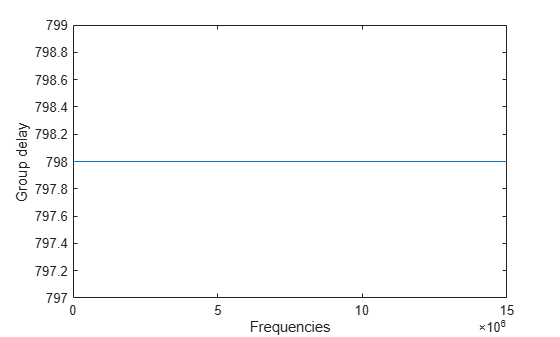# groupDelay

Group delay of digital down converter or digital up converter filter cascade

## Syntax

``D = groupDelay(Conv,N)``
``[D,F] = groupDelay(Conv,N)``

## Description

````D = groupDelay(Conv,N)` returns a vector of group delays, `D`, of a digital down converter or digital up converter, `Conv`, evaluated at `N` frequency points. The frequency points are equally spaced around the upper half of the unit circle. ```

example

````[D,F] = groupDelay(Conv,N)` returns a vector of frequencies, `F`, at which the group delay has been computed. ```

## Examples

collapse all

Compute the group delays of the digital down converter using the `groupDelay` function.

Create a `dsp.DigitalDownConverter` System object with the default settings.

`dwnConv = dsp.DigitalDownConverter`
```dwnConv = dsp.DigitalDownConverter with properties: DecimationFactor: 100 MinimumOrderDesign: true Bandwidth: 200000 StopbandFrequencySource: 'Auto' PassbandRipple: 0.1000 StopbandAttenuation: 60 Oscillator: 'Sine wave' CenterFrequency: 14000000 SampleRate: 30000000 Use get to show all properties ```

Use the `groupDelay` function to compute the vector of group delays. By default, the function evaluates the group delays at 8192 frequency points equally spaced around the upper half of the unit circle.

`D = groupDelay(dwnConv);`

Plot the vector of group delays.

```plot(D) xlabel('Number of frequency points') ylabel('Group delay')```Determine the vector of frequencies over which the group delays are computed and plot them.

```[D,F] = groupDelay(dwnConv); plot(F,D) xlabel('Frequencies') ylabel('Group delay')```## Input Arguments

collapse all

Digital down converter or digital up converter, specified as a `dsp.DigitalDownConverter` or `dsp.DigitalUpConverter` System object™.

Number of frequency points over which the group delays are evaluated, specified as a positive scalar. These points are equally spaced around the upper half of the unit circle.

Data Types: `double` | `single`

## Output Arguments

collapse all

Vector of group delays of the digital down converter or digital up converter, evaluated at `N` frequency points equally spaced around the upper half of the unit circle.

Data Types: `double`

Frequencies at which the group delays are evaluated, returned as a column vector.

Data Types: `double`

## Version History

Introduced in R2012a